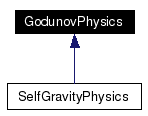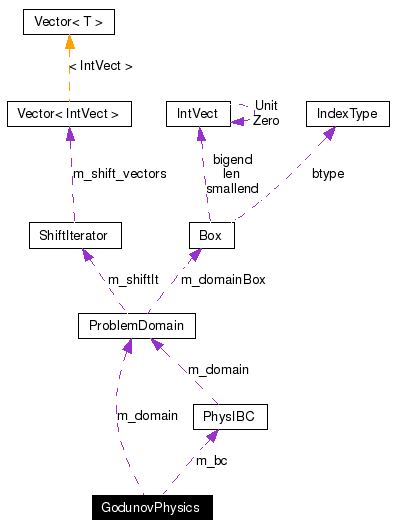# GodunovPhysics Class Reference

`#include <GodunovPhysics.H>`

Inheritance diagram for GodunovPhysics:[legend]
Collaboration diagram for GodunovPhysics:[legend]
List of all members.

## Public Member Functions

GodunovPhysics ()
Constructor.
PhysIBCgetPhysIBC () const
Get the initial and boundary condition object.
void setPhysIBC (PhysIBC *a_bc)
Set the initial and boundary condition object.
virtual ~GodunovPhysics ()
Destructor.
virtual void define (const ProblemDomain &a_domain, const Real &a_dx)
Define the object.
virtual void setCurrentBox (const Box &a_currentBox)
Set the current box (default implementation - do nothing).
virtual Real getMaxWaveSpeed (const FArrayBox &a_U, const Box &a_box)=0
Compute the maximum wave speed.
virtual GodunovPhysicsnew_godunovPhysics () const =0
Object factory for this class.
virtual int numConserved ()=0
Number of conserved variables.
virtual Vector< string > stateNames ()=0
Names of the conserved variables.
virtual int numFluxes ()=0
Number of flux variables.
virtual void getFlux (FArrayBox &a_flux, const FArrayBox &a_whalf, const int &a_dir, const Box &a_box)=0
Compute the fluxes from primitive variable values on a face.
virtual bool isDefined () const
Is the object completely defined.
virtual int numPrimitives ()=0
Number of primitive variables.
virtual void charAnalysis (FArrayBox &a_dW, const FArrayBox &a_W, const int &a_dir, const Box &a_box)=0
Transform a_dW from primitive to characteristic variables.
virtual void charSynthesis (FArrayBox &a_dW, const FArrayBox &a_W, const int &a_dir, const Box &a_box)=0
Transform a_dW from characteristic to primitive variables.
virtual void charValues (FArrayBox &a_lambda, const FArrayBox &a_W, const int &a_dir, const Box &a_box)=0
Compute the characteristic values (eigenvalues).
virtual void incrementSource (FArrayBox &a_S, const FArrayBox &a_W, const Box &a_box)=0
Add to (increment) the source terms given the current state.
virtual void riemann (FArrayBox &a_WStar, const FArrayBox &a_WLeft, const FArrayBox &a_WRight, const FArrayBox &a_W, const Real &a_time, const int &a_dir, const Box &a_box)=0
Compute the solution to the Riemann problem.
virtual void postNormalPred (FArrayBox &a_dWMinus, FArrayBox &a_dWPlus, const FArrayBox &a_W, const Real &a_dt, const Real &a_dx, const int &a_dir, const Box &a_box)=0
Post-normal predictor calculation.
virtual void quasilinearUpdate (FArrayBox &a_AdWdx, const FArrayBox &a_wHalf, const FArrayBox &a_W, const Real &a_scale, const int &a_dir, const Box &a_box)=0
Compute the quasilinear update A*dW/dx.
virtual void consToPrim (FArrayBox &a_W, const FArrayBox &a_U, const Box &a_box)=0
Compute primitive variables from conserved variables.
virtual Interval velocityInterval ()=0
Interval within the primitive variables corresponding to the velocities.
virtual int pressureIndex ()=0
Component index within the primitive variables of the pressure.
virtual Real smallPressure ()=0
Used to limit the absolute value of a "pressure" difference.
virtual int bulkModulusIndex ()=0
Component index within the primitive variables of the bulk modulus.

## Protected Attributes

bool m_isDefined
ProblemDomain m_domain
Real m_dx
PhysIBCm_bc
bool m_isBCSet

## Detailed Description

The base class GodunovPhysics provides the physics-dependent components for a higher-order Godunov method for a single patch: characteristic analysis, Riemann solver, quasilinear update, conservative update, and transformations between conserved, primitive, and flux variables. This class is essentially pure virtual, i.e. all of its member functions are virtual, and the ones that have default implementations are ones which are optionally defined, i.e. the default definition is to send an error message. Physics-dependent versions of this class that are required in real applications are derived from this class by inheritance.

## Constructor & Destructor Documentation

 GodunovPhysics::GodunovPhysics ( )
 Constructor.

 virtual GodunovPhysics::~GodunovPhysics ( ) ` [virtual]`
 Destructor.

## Member Function Documentation

 virtual int GodunovPhysics::bulkModulusIndex ( ) ` [pure virtual]`
 Component index within the primitive variables of the bulk modulus. Return the component index withn the primitive variables for the bulk modulus. Used for slope flattening (slope computation) used as a normalization to measure shock strength. Implemented in SelfGravityPhysics.

 virtual void GodunovPhysics::charAnalysis ( FArrayBox & a_dW, const FArrayBox & a_W, const int & a_dir, const Box & a_box ) ` [pure virtual]`
 Transform a_dW from primitive to characteristic variables. On input, a_dW contains the increments of the primitive variables. On output, it contains the increments in the characteristic variables. IMPORTANT NOTE: It is assumed that the characteristic analysis puts the smallest eigenvalue first, the largest eigenvalue last, and orders the characteristic variables accordingly. Implemented in SelfGravityPhysics.

 virtual void GodunovPhysics::charSynthesis ( FArrayBox & a_dW, const FArrayBox & a_W, const int & a_dir, const Box & a_box ) ` [pure virtual]`
 Transform a_dW from characteristic to primitive variables. On input, a_dW contains the increments of the characteristic variables. On output, it contains the increments in the primitive variables. IMPORTANT NOTE: It is assumed that the characteristic analysis puts the smallest eigenvalue first, the largest eigenvalue last, and orders the characteristic variables accordingly. Implemented in SelfGravityPhysics.

 virtual void GodunovPhysics::charValues ( FArrayBox & a_lambda, const FArrayBox & a_W, const int & a_dir, const Box & a_box ) ` [pure virtual]`
 Compute the characteristic values (eigenvalues). Compute the characteristic values (eigenvalues). IMPORTANT NOTE: It is assumed that the characteristic analysis puts the smallest eigenvalue first, the largest eigenvalue last, and orders the characteristic variables accordingly. Implemented in SelfGravityPhysics.

 virtual void GodunovPhysics::consToPrim ( FArrayBox & a_W, const FArrayBox & a_U, const Box & a_box ) ` [pure virtual]`
 Compute primitive variables from conserved variables. Implemented in SelfGravityPhysics.

 virtual void GodunovPhysics::define ( const ProblemDomain & a_domain, const Real & a_dx ) ` [virtual]`
 Define the object.

 virtual void GodunovPhysics::getFlux ( FArrayBox & a_flux, const FArrayBox & a_whalf, const int & a_dir, const Box & a_box ) ` [pure virtual]`
 Compute the fluxes from primitive variable values on a face. Implemented in SelfGravityPhysics.

 virtual Real GodunovPhysics::getMaxWaveSpeed ( const FArrayBox & a_U, const Box & a_box ) ` [pure virtual]`
 Compute the maximum wave speed. Implemented in SelfGravityPhysics.

 PhysIBC* GodunovPhysics::getPhysIBC ( ) const
 Get the initial and boundary condition object.

 virtual void GodunovPhysics::incrementSource ( FArrayBox & a_S, const FArrayBox & a_W, const Box & a_box ) ` [pure virtual]`
 Add to (increment) the source terms given the current state. On input, a_S contains the current source terms. On output, a_S has had any additional source terms (based on the current state, a_W) added to it. This should all be done on the region defined by a_box. Implemented in SelfGravityPhysics.

 virtual bool GodunovPhysics::isDefined ( ) const` [virtual]`
 Is the object completely defined. Return true if the object is completely defined.

 virtual GodunovPhysics* GodunovPhysics::new_godunovPhysics ( ) const` [pure virtual]`
 Object factory for this class. Implemented in SelfGravityPhysics.

 virtual int GodunovPhysics::numConserved ( ) ` [pure virtual]`
 Number of conserved variables. Return the number of conserved variables. Implemented in SelfGravityPhysics.

 virtual int GodunovPhysics::numFluxes ( ) ` [pure virtual]`
 Number of flux variables. Return the number of flux variables. This can be greater than the number of conserved variables if addition fluxes/face-centered quantities are computed. Implemented in SelfGravityPhysics.

 virtual int GodunovPhysics::numPrimitives ( ) ` [pure virtual]`
 Number of primitive variables. Return the number of primitive variables. This may be greater than the number of conserved variables if derived/redundant quantities are also stored for convenience. Implemented in SelfGravityPhysics.

 virtual void GodunovPhysics::postNormalPred ( FArrayBox & a_dWMinus, FArrayBox & a_dWPlus, const FArrayBox & a_W, const Real & a_dt, const Real & a_dx, const int & a_dir, const Box & a_box ) ` [pure virtual]`
 Post-normal predictor calculation. Add increment to normal predictor, e.g. to account for source terms due to spatially-varying coefficients, to bound primitive variable ranges. Implemented in SelfGravityPhysics.

 virtual int GodunovPhysics::pressureIndex ( ) ` [pure virtual]`
 Component index within the primitive variables of the pressure. Return the component index withn the primitive variables for the pressure. Used for slope flattening (slope computation). Implemented in SelfGravityPhysics.

 virtual void GodunovPhysics::quasilinearUpdate ( FArrayBox & a_AdWdx, const FArrayBox & a_wHalf, const FArrayBox & a_W, const Real & a_scale, const int & a_dir, const Box & a_box ) ` [pure virtual]`
 Compute the quasilinear update A*dW/dx. Implemented in SelfGravityPhysics.

 virtual void GodunovPhysics::riemann ( FArrayBox & a_WStar, const FArrayBox & a_WLeft, const FArrayBox & a_WRight, const FArrayBox & a_W, const Real & a_time, const int & a_dir, const Box & a_box ) ` [pure virtual]`
 Compute the solution to the Riemann problem. Given input left and right states in a direction, a_dir, compute a Riemann problem and generate fluxes at the faces within a_box. Implemented in SelfGravityPhysics.

 virtual void GodunovPhysics::setCurrentBox ( const Box & a_currentBox ) ` [virtual]`
 Set the current box (default implementation - do nothing).

 void GodunovPhysics::setPhysIBC ( PhysIBC * a_bc )
 Set the initial and boundary condition object.

 virtual Real GodunovPhysics::smallPressure ( ) ` [pure virtual]`
 Used to limit the absolute value of a "pressure" difference. Return a value that is used by slope flattening to limit (away from zero) the absolute value of a slope in the pressureIndex() component (slope computation). Implemented in SelfGravityPhysics.

 virtual Vector GodunovPhysics::stateNames ( ) ` [pure virtual]`
 Names of the conserved variables. Return the names of the conserved variables. Implemented in SelfGravityPhysics.

 virtual Interval GodunovPhysics::velocityInterval ( ) ` [pure virtual]`
 Interval within the primitive variables corresponding to the velocities. Return the interval of component indices within the primitive variable of the velocities. Used for slope flattening (slope computation) and computing the divergence of the velocity (artificial viscosity). Implemented in SelfGravityPhysics.

## Member Data Documentation

 PhysIBC* GodunovPhysics::m_bc` [protected]`

 ProblemDomain GodunovPhysics::m_domain` [protected]`

 Real GodunovPhysics::m_dx` [protected]`

 bool GodunovPhysics::m_isBCSet` [protected]`

 bool GodunovPhysics::m_isDefined` [protected]`

The documentation for this class was generated from the following file:
Generated on Wed Oct 5 14:00:55 2005 for Chombo&AMRSelfGravity by1.4.1